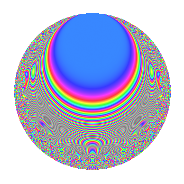Properties

 Label 1002.2.dLevel 1002 Weight 2 Character orbit d Rep. character $$\chi_{1002}(1001,\cdot)$$ Character field $$\Q$$ Dimension 56 Newforms 1 Sturm bound 336 Trace bound 0

Related objects

Defining parameters

 Level: $$N$$ = $$1002 = 2 \cdot 3 \cdot 167$$ Weight: $$k$$ = $$2$$ Character orbit: $$[\chi]$$ = 1002.d (of order $$2$$ and degree $$1$$) Character conductor: $$\operatorname{cond}(\chi)$$ = $$501$$ Character field: $$\Q$$ Newforms: $$1$$ Sturm bound: $$336$$ Trace bound: $$0$$

Dimensions

The following table gives the dimensions of various subspaces of $$M_{2}(1002, [\chi])$$.

Total New Old
Modular forms 172 56 116
Cusp forms 164 56 108
Eisenstein series 8 0 8

Trace form

 $$56q$$ $$\mathstrut -\mathstrut 56q^{4}$$ $$\mathstrut +\mathstrut 4q^{6}$$ $$\mathstrut +\mathstrut 4q^{9}$$ $$\mathstrut +\mathstrut O(q^{10})$$ $$56q$$ $$\mathstrut -\mathstrut 56q^{4}$$ $$\mathstrut +\mathstrut 4q^{6}$$ $$\mathstrut +\mathstrut 4q^{9}$$ $$\mathstrut +\mathstrut 56q^{16}$$ $$\mathstrut +\mathstrut 8q^{18}$$ $$\mathstrut +\mathstrut 8q^{19}$$ $$\mathstrut +\mathstrut 16q^{21}$$ $$\mathstrut -\mathstrut 4q^{24}$$ $$\mathstrut +\mathstrut 64q^{25}$$ $$\mathstrut +\mathstrut 12q^{27}$$ $$\mathstrut +\mathstrut 16q^{31}$$ $$\mathstrut +\mathstrut 40q^{33}$$ $$\mathstrut -\mathstrut 4q^{36}$$ $$\mathstrut -\mathstrut 20q^{42}$$ $$\mathstrut +\mathstrut 32q^{49}$$ $$\mathstrut -\mathstrut 16q^{54}$$ $$\mathstrut +\mathstrut 36q^{57}$$ $$\mathstrut +\mathstrut 16q^{61}$$ $$\mathstrut +\mathstrut 16q^{63}$$ $$\mathstrut -\mathstrut 56q^{64}$$ $$\mathstrut +\mathstrut 8q^{66}$$ $$\mathstrut -\mathstrut 8q^{72}$$ $$\mathstrut -\mathstrut 24q^{75}$$ $$\mathstrut -\mathstrut 8q^{76}$$ $$\mathstrut +\mathstrut 36q^{81}$$ $$\mathstrut -\mathstrut 16q^{84}$$ $$\mathstrut -\mathstrut 64q^{85}$$ $$\mathstrut -\mathstrut 4q^{87}$$ $$\mathstrut +\mathstrut 64q^{93}$$ $$\mathstrut -\mathstrut 40q^{94}$$ $$\mathstrut +\mathstrut 4q^{96}$$ $$\mathstrut -\mathstrut 72q^{97}$$ $$\mathstrut -\mathstrut 64q^{99}$$ $$\mathstrut +\mathstrut O(q^{100})$$

Decomposition of $$S_{2}^{\mathrm{new}}(1002, [\chi])$$ into irreducible Hecke orbits

Label Dim. $$A$$ Field CM Traces $q$-expansion
$$a_2$$ $$a_3$$ $$a_5$$ $$a_7$$
1002.2.d.a $$56$$ $$8.001$$ None $$0$$ $$0$$ $$0$$ $$0$$

Decomposition of $$S_{2}^{\mathrm{old}}(1002, [\chi])$$ into lower level spaces

$$S_{2}^{\mathrm{old}}(1002, [\chi]) \cong$$ $$S_{2}^{\mathrm{new}}(501, [\chi])$$$$^{\oplus 2}$$